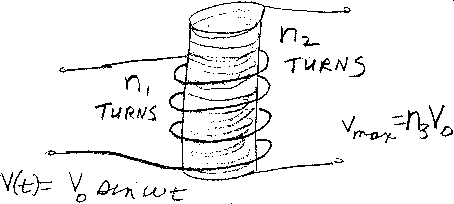Problem C10: Consider the transformer shown in the figure above. The first coil has n1 turns of wire, and the second coil has n2 turns of wire. The voltage across the first coil is V(t) = V0 sin (ωt) . What is the magnitude of the maximum voltage induced on the second coil? That is, if |Vmax| = n3 V0, what is n3?n1 = n2 = Input n3:
If you are currently in my class, you can record your grade by entering your name and student ID number (without the leading zeros) below and clicking on "record grade".
 First Name = Last Name = ID = Problem: